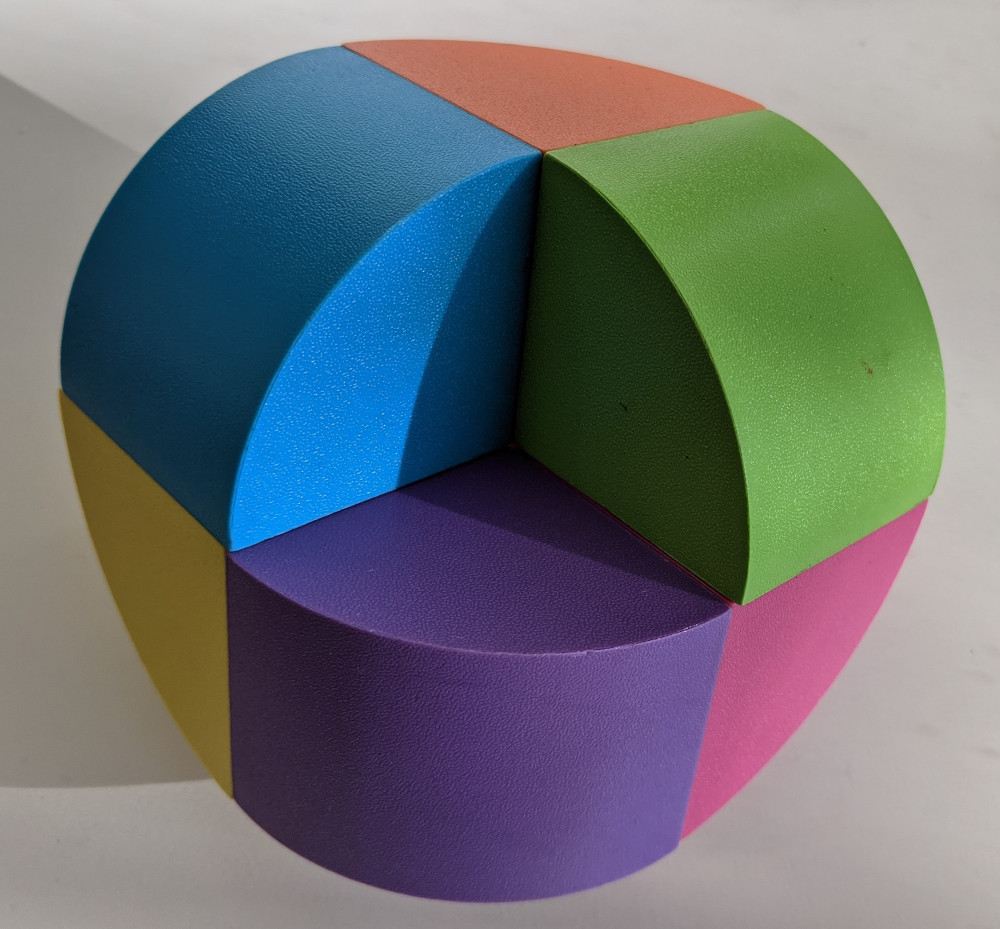# teaching machines

## Tricube

November 1, 2020 by . Filed under madeup, public.

A month ago one of my sons’ toys inspired me to try and model it in Madeup. Here is the original set of magnetic blocks:And here is my first attempt at modeling them using Madeup’s dowel command with rounded bends:



moveto(x = 0, y = 0)

repeat 3
move(distance = 1)
yaw(degrees = 90)
move(distance = 1)
pitch(degrees = -90)

dowel(sharpness = 5)

var mupDiv = jQuery('#mup_shape');
mupDiv.closest('pre').replaceWith(mupDiv);
document.getElementById('mup_form_shape').submit();

moveto(x = 0, y = 0)

repeat 3
move(distance = 1)
yaw(degrees = 90)
move(distance = 1)
pitch(degrees = -90)

dowel(sharpness = 5)



Notice the hole in the middle. Given how the rounding is implemented, I was not able to remove it. You can bet that I was haunted by that hole. I took some time to design a new solidifier so that I could create this shape. The solidifier is called table. One feeds it a list of cross sections, all of the same cardinality, and the cross sections are connected together.

The shape generated by table has no hole.



moveto(x = 0, y = 0)
pitch(degrees = -90)
repeat 4
move(distance = 1)
yaw(degrees = -90)

path = mold()
paths = [path]

n = 20
degrees = 90.0 / n

axes = [:backward, :up, :right]

repeat 2
for axis of axes
repeat n
path = rotate(path, degrees, axis)
paths.push(item = path)

table(rows = paths)

var mupDiv = jQuery('#mup_tricube');
mupDiv.closest('pre').replaceWith(mupDiv);
document.getElementById('mup_form_tricube').submit();

moveto(x = 0, y = 0)
pitch(degrees = -90)
repeat 4
move(distance = 1)
yaw(degrees = -90)

path = mold()
paths = [path]

n = 20
degrees = 90.0 / n

axes = [:backward, :up, :right]

repeat 2
for axis of axes
repeat n
path = rotate(path, degrees, axis)
paths.push(item = path)

table(rows = paths)



Someday soon I’ll have a more general loft solidifier, which will allow cross sections of different cardinalities.